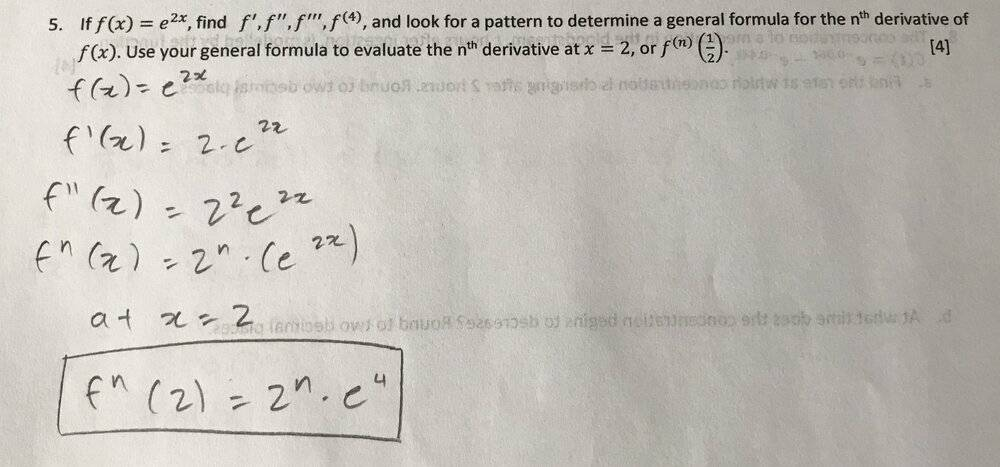# Solving for f',f'',f''' and determining a general formula

Homework Statement:
Can someone confirm if my work is correct?
Relevant Equations:
n/aHallsofIvy
Homework Helper
I'm a bit surprised you did not do what you were asked to do! The problem specifically asked you to "find $f'(x)$, $f''(x)$, $f'''(x)$, and $f^{IV}(x)$ then the nth derivative but you have only found $f'(x)$ and $f''(x)$ before jumping to the nth derivative.
Other than the fact that you do not have $f'''(x)= 2^3 e^{2x}$ and $f^{IV}(x)= 2^4e^{2x}$, every thing is good!

•roam
benorin
Homework Helper
The problem also mentions "or ##f^{(n)}\left( \tfrac{1}{2}\right)##", which is of course ##\boxed{f^{(n)}\left( \tfrac{1}{2}\right) = 2^n e}##.